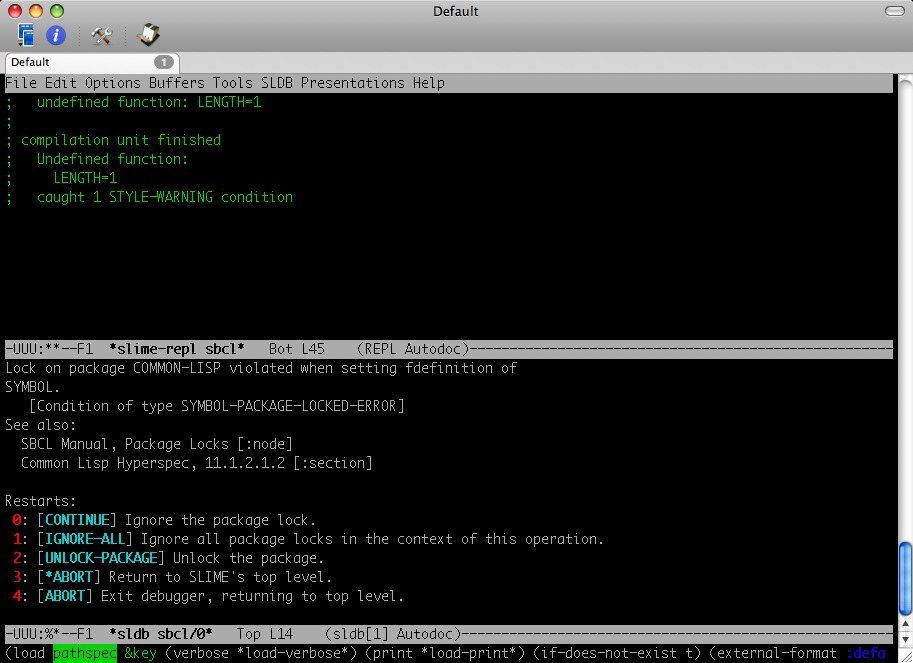# Drake's Weblog

## Lisp 初體驗：Grammar Induction

Anyway，借用一個這樣的 random stuff generator 來找點子，實在太冒險也太不明智了，況且，我還處於 non-qualified Ph.D. student，沒啥理由理會這個網站的。除非…

ok, 首先，我一點都不懂 AI, NLP. 就算有修過一堂 AI 課，也是以非常低分，只比及格還來得高一點的方式過關。（沒辦法，大二在什麼都不懂的情況下，就跟著同學起鬨去修了許永貞教授的課。我還記得那是堂限定大三以上才可以修的課，但我們一群人居然表現出無比的勇氣與真誠，感動了教授，於是我在懞懂中修完了 ＡＩ，至今還是覺得很後悔，應該大四再去修，收獲比較完整的）加上我也沒看過 ＰＡＩＰ 這本書，一開始想以最快最省事的方式，把玩一下先。

• 下載 PAIP 程式碼，解開至某個資料夾。

• 執行 Emacs interactive shell（或是採用 Emacs + SLIME），根據原網頁上寫的，先載入 auxfns 這個檔

``````        (load "auxfns.lisp")
``````
• 有很高的機會，你會看到如下的一些畫面，持續按 0 (continue) 就對了。（原因是新的 Lisp interpreter 已經內建了 symbol 與 debug 這兩個函式了）• 麻煩的事來了。接著是按照網頁上的建議，想執行

``````        (requires "examples")
``````

• 這是 auxfns.lisp 裏的 load-paip-file 這個函式的問題。它原來的程式碼如下：

``````        (defun load-paip-file (file)
``````

“Load the binary file if it exists and is newer, else load the source.” (let* ((src (paip-pathname file :lisp)) (src-date (file-write-date src)) (bin (paip-pathname file :binary)) (bin-date (file-write-date bin))) (load (if (and (probe-file bin) src-date bin-date (>= bin-date src-date)) bin src))))

``````            (defun load-paip-file (file)
``````
• 最後，我們重新來一次，順利執行過了 (requires “examples”)，接著輸入

``````        (do-examples :all)
``````

``````(defparameter *grammar4*
'((NP -> (D N))
(NP -> (N))
(NP -> (D A+ N))
(NP -> (A+ N))
(NP -> (NP PP))
(PP -> (P NP))
(N -> (N N))
(A+ -> (A))
(A+ -> (A A+))
(A -> abstract) (A -> adaptive) (A -> anisotropic) (A -> arbitrary) (A -> ambient)
(A -> bidirectional)
(A -> distributed) (A -> dynamic)
(A -> exponential)
(A -> gaussian)
(A -> hardware-accelerated) (A -> hierarchical)
(A -> intelligent) (A -> interactive) (A -> inverse)
(A -> linear) (A -> logarithmic)
(A -> multi-resolution)
(A -> non-linear) (A -> normal) (A -> normal-mapped)
(A -> occlusion-mapped) (A -> omnidirectional) (A -> output-sensitive)
(A -> parameterized) (A -> persistent) (A -> piecewise) (A -> polygonal) (A -> practical) (A -> pre-computed) (A -> precise) (A -> probabalistic) (A -> procedural)
(A -> real-time) (A -> reflective) (A -> relief)
(A -> shadow-mapped) (A -> soft) (A -> spherical)
(A -> texture-mapped)
(A -> variable) (A -> view-dependent) (A -> volumetric)
(D -> the) (D -> a) (D -> an)
(N -> approximation) (N -> attenuation)
(N -> caustics) (N -> clustering) (N -> compression) (N -> culling)
(N -> displacement)
(N -> estimation)
(N -> fields)
(N -> geometry)
(N -> interpolation)
(N -> light)
(N -> mapping) (N -> map) (N -> mipmap) (N -> modeling)
(N -> occlusion) (N -> opacity)
(N -> preprocessing)
(N -> re-meshing) (N -> rendering)
(N -> sampling) (N -> shadow) (N -> spline) (N -> subsurface scattering) (N -> surface)
(N -> texture) (N -> texturing)
(P -> with) (P -> for) (P -> at) (P -> on) (P -> by) (P -> of) (P -> in) (P -> using)
))
``````

• None